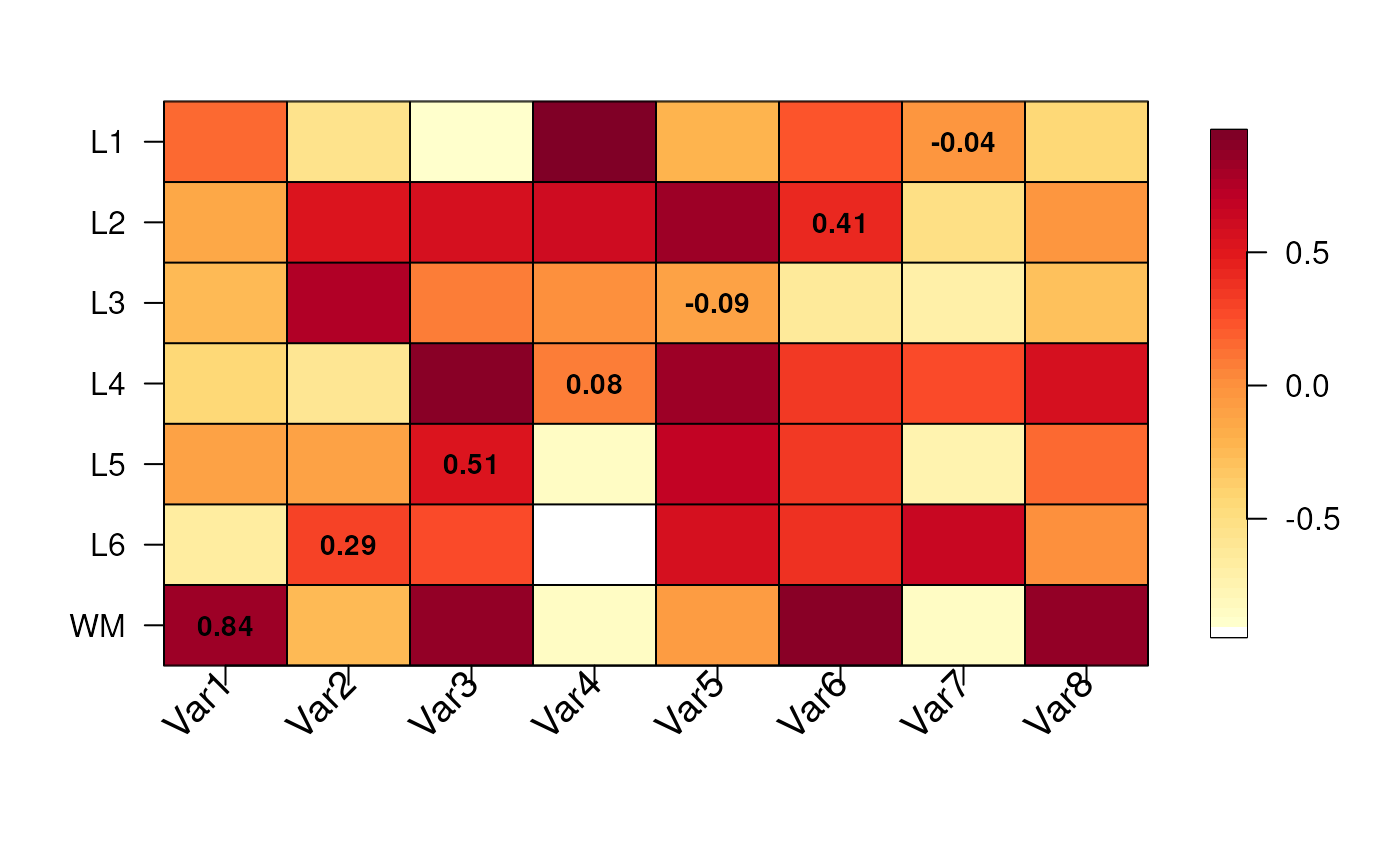This function visualizes a numerical matrix where the Y-axis represents the human brain layers and can be adjusted to represent the length of each brain layer. Cells can optionally have text values. This function is used by gene_set_enrichment_plot() and layer_stat_cor_plot().

layer_matrix_plot(
matrix_values,
matrix_labels = NULL,
xlabs = NULL,
layerHeights = NULL,
mypal = c("white", (grDevices::colorRampPalette(RColorBrewer::brewer.pal(9,
"YlOrRd")))(50)),
breaks = NULL,
axis.args = NULL,
srt = 45,
mar = c(8, 4 + (max(nchar(rownames(matrix_values)))%/%3) * 0.5, 4, 2) + 0.1,
cex = 1.2
)

## Arguments

matrix_values

A matrix() with one column per set of interest and one row per layer (group) with numeric values.

matrix_labels

Optionally a character matrix() with the same dimensions and dimnames() as matrix_values with text labels for the cells.

xlabs

A vector of names in the same order and length as colnames(matrix_values).

layerHeights

A numeric() vector of length equal to nrow(matrix_values) + 1 that starts at 0 specifying where to plot the y-axis breaks which can be used for re-creating the length of each brain layer.

mypal

A vector with the color palette to use.

breaks

Passed to fields::image.plot(). Used by layer_stat_cor_plot().

axis.args

Passed to fields::image.plot(). Used by layer_stat_cor_plot().

srt

The angle for the x-axis labels. Used by layer_stat_cor_plot().

mar

Passed to graphics::par().

cex

Used for the x-axis labels and the text inside the cells.

## Value

A base R plot visualizing the input matrix_values with optional text labels for matrix_labels.

## Examples


## Create some random data
set.seed(20200224)
mat <- matrix(runif(7 * 8, min = -1), nrow = 7)
rownames(mat) <- c("WM", paste0("L", rev(seq_len(6))))
colnames(mat) <- paste0("Var", seq_len(8))

## Create some text labels
mat_text <- matrix("", nrow = 7, ncol = 8, dimnames = dimnames(mat))
diag(mat_text) <- as.character(round(diag(mat), 2))

## Make the plot
layer_matrix_plot(mat, mat_text)## Try to re-create the anatomical proportions of the human brain layers
layer_matrix_plot(
mat,
mat_text,
layerHeights = c(0, 40, 55, 75, 85, 110, 120, 135),
cex = 2
)